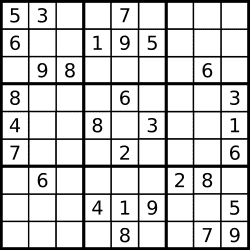36. Valid Sudoku 验证数独

Determine if a 9x9 Sudoku board is valid. Only the filled cells need to be validated according to the following rules:

Each row must contain the digits 1-9 without repetition. Each column must contain the digits 1-9 without repetition. Each of the 9 3x3 sub-boxes of the grid must contain the digits 1-9 without repetition.The Sudoku board could be partially filled, where empty cells are filled with the character ‘.’.

Note:

A Sudoku board (partially filled) could be valid but is not necessarily solvable. Only the filled cells need to be validated according to the mentioned rules. The given board contain only digits 1-9 and the character ‘.’. The given board size is always 9x9.

Difficulty: Medium

class Solution {
public:
bool isValidSudoku(vector<vector<char>>& board) {
if (board.empty()||board.empty()) return false;
int m = board.size(),n = board.size();
vector<vector<bool>> rowFlag(m,vector<bool>(n,false));
vector<vector<bool>> colFlag(m,vector<bool>(n,false));
vector<vector<bool>> cellFlag(m,vector<bool>(n,false));
for (int i=0;i<m;++i){
for (int j=0; j<n; ++j) {
if (board[i][j]>='1'&&board[i][j]<='9'){
int c = board[i][j]-'1';
if (rowFlag[i][c] || colFlag[c][j] || cellFlag[3*(i/3) + j/3][c])
{
return false;
}
rowFlag[i][c] = true;
colFlag[c][j] = true;
cellFlag[3*(i/3) + j/3][c] = true;
}
}
}
return  true;
}
};